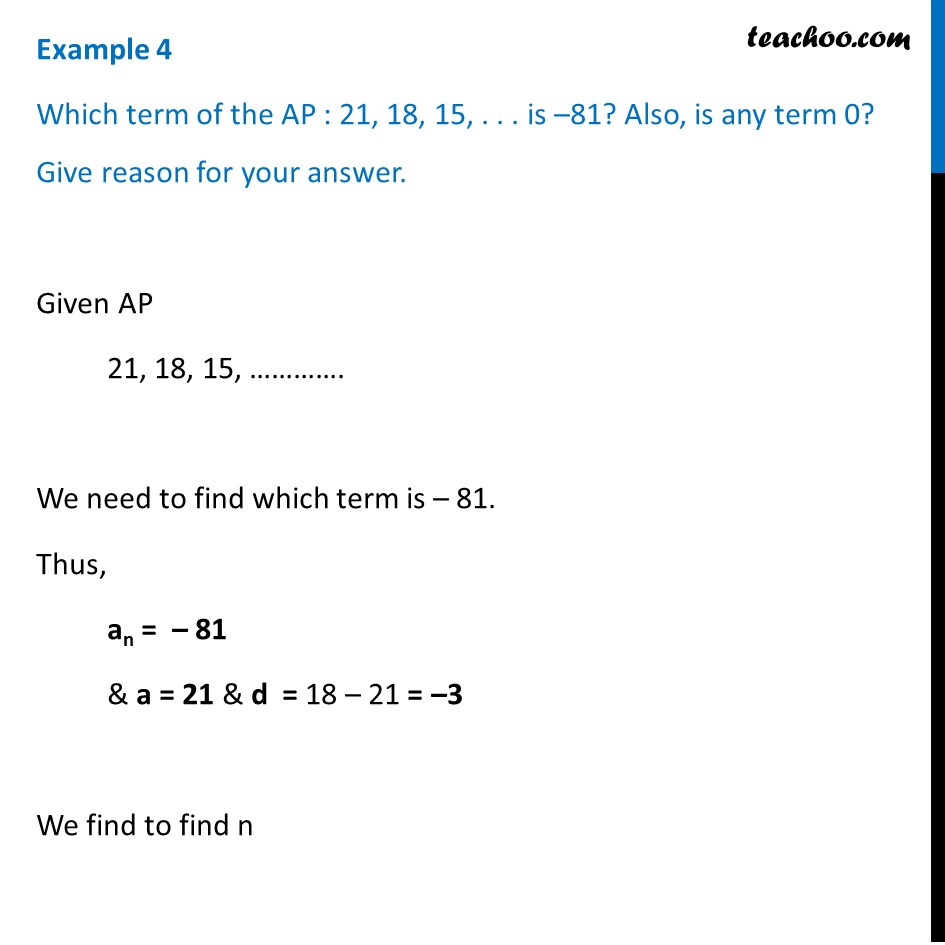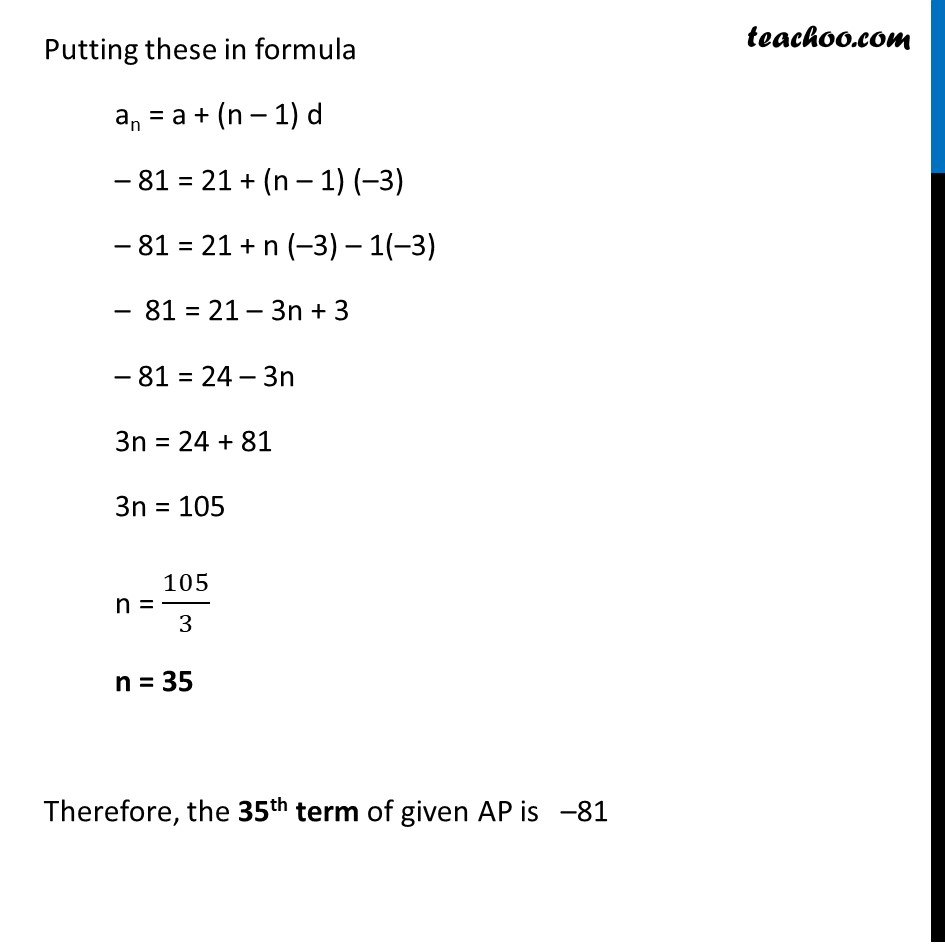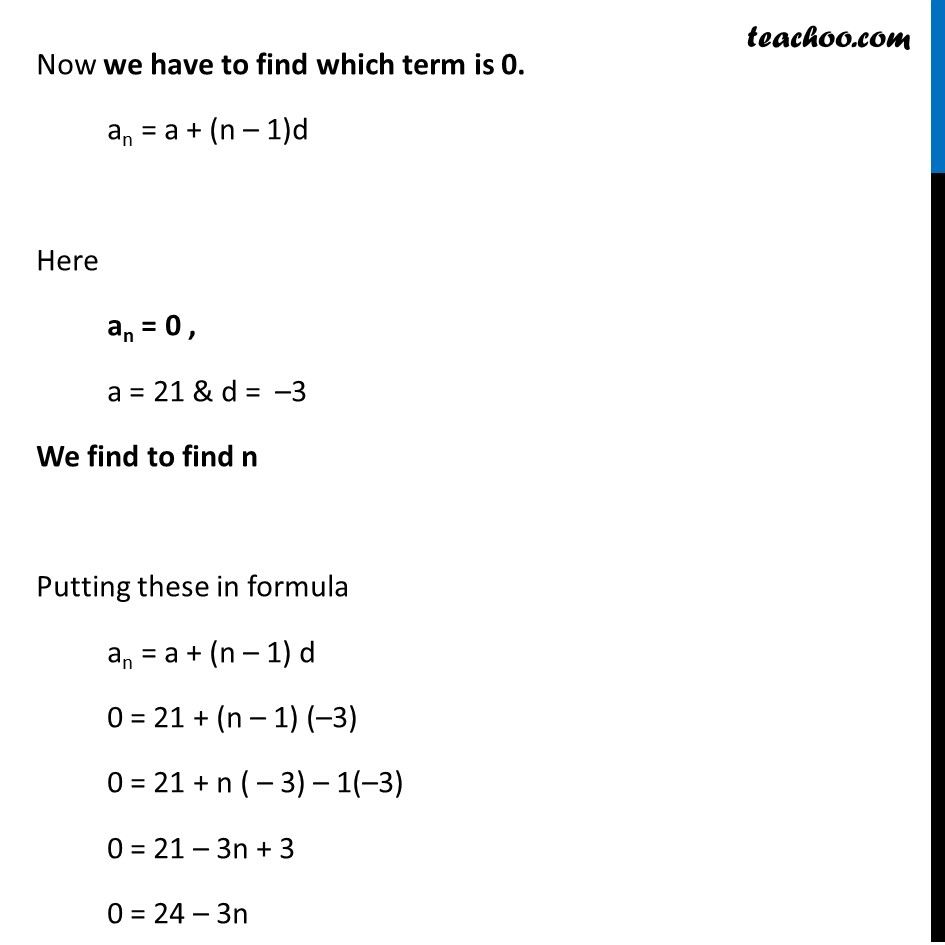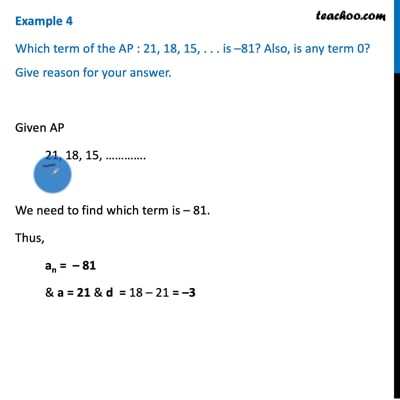Finding n

Chapter 5 Class 10 Arithmetic Progressions (Term 2)
Concept wiseThis video is only available for Teachoo black users

### Transcript

Example 4 Which term of the AP : 21, 18, 15, . . . is –81? Also, is any term 0? Give reason for your answer. Given AP 21, 18, 15, …………. We need to find which term is – 81. Thus, an = – 81 & a = 21 & d = 18 – 21 = –3 We find to find n Putting these in formula an = a + (n – 1) d – 81 = 21 + (n – 1) (–3) – 81 = 21 + n (–3) – 1(–3) – 81 = 21 – 3n + 3 – 81 = 24 – 3n 3n = 24 + 81 3n = 105 n = 105/3 n = 35 Therefore, the 35th term of given AP is –81 Now we have to find which term is 0. an = a + (n – 1)d Here an = 0 , a = 21 & d = –3 We find to find n Putting these in formula an = a + (n – 1) d 0 = 21 + (n – 1) (–3) 0 = 21 + n ( – 3) – 1(–3) 0 = 21 – 3n + 3 0 = 24 – 3n 3n = 24 n = 24/3 n = 8 Therefore, the 8th term of given AP is 0,